Courses

# Test: Permutation, Combination & Probability

## 10 Questions MCQ Test Topic-wise Past Year Questions for CAT | Test: Permutation, Combination & Probability

Description
This mock test of Test: Permutation, Combination & Probability for CAT helps you for every CAT entrance exam. This contains 10 Multiple Choice Questions for CAT Test: Permutation, Combination & Probability (mcq) to study with solutions a complete question bank. The solved questions answers in this Test: Permutation, Combination & Probability quiz give you a good mix of easy questions and tough questions. CAT students definitely take this Test: Permutation, Combination & Probability exercise for a better result in the exam. You can find other Test: Permutation, Combination & Probability extra questions, long questions & short questions for CAT on EduRev as well by searching above.
QUESTION: 1

### How many numbers with two or more digits can be formed with the digits 1,2,3,4,5,6,7,8,9, so that in every such number, each digit is used at most once and the digits appear in the ascending order? (2018)

Solution:

As the digits appear in ascending order in the numbers, number of ways of forming a n-digit number using the 9 digits = 9Cn
Number of possible two-digit numbers which can be formed
= 9C2 + 9C3 + 9C4 + 9C5 + 9C6 + 9C7 + 9C8 + 9C9
= (1 + 1)9 – (9C0 + 9C1) = 29 – (9C0 + 9C1) = 512 – (1 + 9) = 502

QUESTION: 2

### The first n natural numbers, 1 to n, have to be arranged in a row from left to right. The n numbers are arranged such that there are an odd number of numbers between any two even numbers as well as between any two odd numbers. If the number of ways in which this can be done is 72, then find the value of n. (2016)

Solution:

If n is even, i.e., say n =  2 m then the number of ways is 2 × m! × m!, i.e., m odd numbers in alternate places and m even numbers in alternate places.
If n is odd, i.e., say n = 2m + 1, then the number of ways = m!(m + 1)!
Hence, either 2(m!)2 = 72 or m! (m + 1)! = 72 If 2(m!)2 = 72 ⇒ m! = 6 ⇒ m = 3
for m!(m + 1)! = 72, there is no solution.
Hence m = 3, and n = 2m = 6.

QUESTION: 3

### If we arrange the letters of the word ‘KAKA’ in all possible ways, what is the probability that vowels will not be together in an arrangement? (2015)

Solution:

Letters of the word 'KAKA' can be arranged in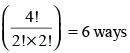If we will take all vowels are together, then arrangements are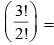3 arrangements.
So, (6 – 3) = 3 arrangements, where vowels are not together.
So, required probability =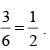QUESTION: 4

Three persons - A, B and C - are playing the game of death. 3 bullets are placed randomly in a revolver having 6 chambers. Each one has to shoot himself by pulling the trigger once after which the revolver passes to the next person. This process continues till two of them are dead and the survivor of the game becomes the winner. What is the probability that B is the winner if A starts the game and A, B and C take turns in that order.

(2015)

Solution:

Total number of the cases = 6C3 = 20.
The favourable cases for B surviving are: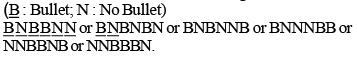Required  probability = 6/20 = 0.3.

QUESTION: 5

Amar, Akbar and Antony are three students in a class of 9 students. A class photo is taken. The number of ways in which it can be taken such that no two of Amar, Akbar and Antony are sitting together is:

(2014)

Solution:

First let the 6 other students be seated in 6 chairs.
The number of spaces between the 6 students = 7.
∴ Amar, Akbar and Anthony can be seated in the 7 places in 7C3 ways.
Thus, the number of ways in the class photo can be taken such that no two of Amar, Akbar and Anthony are sitting together is
= 7C3 × 3! × 6! = 151200.

QUESTION: 6

If p is the probability of head turning up in the toss of a coin (not necessarily fair) and q is the probability of a tail turning up. Find the minimum possible value of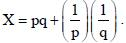(2014)

Solution:

p + q = 1, i.e. q = 1 – p (0 = p, q = 1)
Now when the sum of two variables is a constant then their multiplication is the maximum when they are equal.
So, pq will be maximum and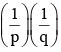the
minimum when p = q = 1/2.
Thus, the minimum value of X  = 0.25 + 4 = 4.25.

QUESTION: 7

There are exactly sixty chairs around a circular table. There are some people sitting on these chairs in such a way that the next person to be seated around the table will have to sit next to someone. What is the least possible number of people sitting around the table currently?

(2014)

Solution:

If there are 60 chairs around a circular table, consider a scenario wherein there ae two chairs vacant between every two consecutive people. Thus, there will be exactly 20 people sitting and exactly 40 vacant seats between them and in such a scenario, next person coming to sit will have to sit next to someone.

QUESTION: 8

In how many ways can 18 identical candies be distributed among 8 children such that the number of candies received by each child is a prime number?

(2013)

Solution:

Since the number of candies received by each child must get at least 2 candies.
Once each child has received 2 candies, the remaining 2 candies should be distributed in such a maniner that the number of candies with any child after the distribution remains a prime number.
The remaining 2 candies are given to exactly two children in such a way that both the children receive one candy each.
Hence, the number of ways of distribution = 8C2 = 28.

QUESTION: 9

What is the probability that the product of two integers chosen at random has the same unit digit as the two integers?

(2012)

Solution:

An integer can end with any of ten digits = (0, 1, 2, ......9) but (0, 1, 5, 6) has same unit of ten product of two integers.
So, the probability of an integer ending with 0 or 1 or 5 or 6.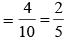and the probability of 2nd integer = 1/10
∴  Required probability =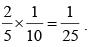QUESTION: 10

A box contains five yellow and five green balls. A ball is picked from the box and is replaced by a ball of the other colour. For instance, if a green ball is picked then it is replaced by a yellow ball and vice-versa. The process is repeated ten times and then a ball is picked from the box. What is the probability that this ball is yellow?

(2011)

Solution:

The number of yellow balls initially in the box is the same as the number of green balls. None of the ten operations involved after this favour any particular colour between the two. So the probability of the final ball picked being yellow (p(Y)) must be the same as that of being green (p(G)).
Thus, p(Y) = p(G) and the ball picked should be either yellow or green, which means that p(Y) + p(G) = 1.
Hence, p(Y) = p(G) = 1/2